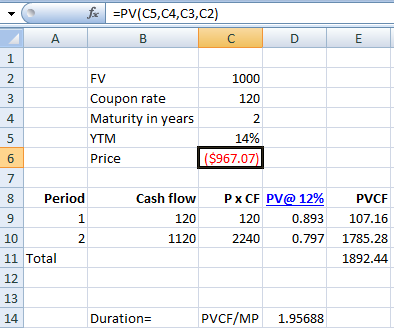# What is the duration of a two-year bond that pays an annual coupon of 12 percent and has a...

## Question:

What is the duration of a two-year bond that pays an annual coupon of 12 percent and has a current yield to maturity of 14 percent? Use $1,000 as the face value. (Do not round intermediate calculations. Round your answer to 4 decimal places. (e.g., 32.1616)) b. What is the duration of a two-year zero-coupon bond that is yielding 11.5 percent? Use$1,000 as the face value.

## Duration of bond

A bond's duration is a measure of its sensitivity to interest rate changes. Higher is the bond's duration, the greater sensitivity to change it implies (which adds to its volatility) and vice versa.

a.

First we calculate the Market price of the bond in excel:The usual Macaulay's duration formula is very complicated. We can calculate this...

Become a Study.com member to unlock this answer! Create your account